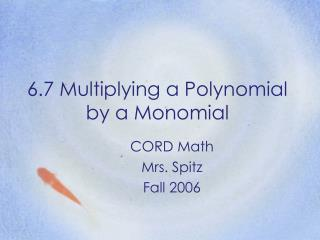DownloadDownload Presentation6.7 Multiplying a Polynomial by a Monomial

6.7 Multiplying a Polynomial by a Monomial

Télécharger la présentation6.7 Multiplying a Polynomial by a Monomial

- - - - - - - - - - - - - - - - - - - - - - - - - - - E N D - - - - - - - - - - - - - - - - - - - - - - - - - - -
Presentation Transcript

1. 6.7 Multiplying a Polynomial by a Monomial CORD Math Mrs. Spitz Fall 2006

2. Objectives: • After studying this lesson, you should be able to: • Multiply a polynomial by a monomial, and • Simplify expressions involving polynomials

3. Assignment • 6.7 Worksheet

4. Application • The world’s largest swimming pool is the Orthlieb Pool in Casablanca, Morocco. It is 30 meters longer than 6 times its width. Express the area of the swimming pool algebraically. • To find the area of the swimming pool, multiply the length by the width. Let w represent the width. Then 6w + 30 represents the length.

5. This diagram of the swimming pool shows that the area is w(6w + 30) square meters. This diagram of the same swimming pool shows that the area is (6w2 + 30w) square meters. What does this look like? 30 6w + 30 6w A = 30w w A = w(6w + 30) w A = 6w2 • Since the areas are equal, w(6w + 30) = 6w2 + 30w • The application above shows how the distributive property can be used to multiply a polynomial by a monomial.

6. A. Use the distributive property. 5a(3a2 + 4) = 5a(3a2) + 5a(4) = 15a3 + 20a B. Multiply each term by 5a. 3a2 + 4 (x) 5a 15a3 + 20a Ex. 1: Find 5a(3a2 + 4)You can multiply either horizontally or vertically.

7. Ex. 2: Find 2m2(5m2 – 7m + 8) Use the distributive property. 2m2(5m2 – 7m + 8) = 2m2(5m2) + 2m2(-7m) + 2m2(8) = 10m4 - 14m3 + 16m2 Ex. 3: Find -3xy(2x2y + 3xy2 – 7y3). Use the distributive property. -3xy (2x2y + 3xy2 – 7y3) = -3xy(2x2y) + (-3xy)(3xy2)+(-3xy)(-7y3). = - 6x3y2 - 9x2y3 + 21xy4

8. Ex. 4: Find the measure of the area of the shaded region in simplest terms. 5a2 + 3a - 2 = 2a(5a2 + 3a – 2) - 8(3a2 – 7a + 1 = 10a3 + 6a2 – 4a - 24a2 + 56a – 8 = 10a3 - 18a2 + 52a – 8 The measure of the area of the shaded region is 10a3 - 18a2 + 52a – 8. 3a2 - 7a + 1 2a 8

9. 7 10 Ex. 5: Many equation contain polynomials that must be added, subtracted, or multiplied before the equation is solved. Solve x(x – 3) + 4x – 3 = 8x + 4 + x (3 + x) x(x – 3) + 4x – 3 = 8x + 4 + x (3 + x) x2 – 3x + 4x – 3 = 8x + 4 + 3x + x2 Multiply x2 + x – 3 = x2 + 11x + 4 Combine like terms x – 3 = 11x + 4 Subtract x2 from each side. - 3 = 10x + 4 Subtract x from each side. - 7 = 10x Subtract 4 from each side. - = x Check this result.